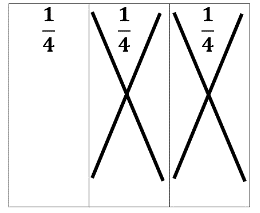# Access Point #: MAFS.4.NF.2.AP.3c (Archived Access Point)

This document was generated on CPALMS - www.cpalms.org

Solve word problems involving addition and subtraction of fractions with like denominators (2, 3, 4 or 8).

#### Essential Understandings

Concrete:

• Match the vocabulary in a word problem to an action.
• Use manipulatives to model the context of the word problem.
• Count to find the answer.
• To add, use fraction manipulatives (each piece may be labeled with the corresponding unit fraction) to model each fraction and join them to find the sum (e.g.,1/4 + 2/4 = 3/4).
• To subtract, use fraction manipulatives (each piece may be labeled with the corresponding unit fraction) to model the first fraction in the expression and remove manipulatives that represent the fraction being subtracted (e.g., 3/4 – 2/4 = 1/4 ).Representation:
• Create a pictorial representation of the word problem.
• Use context clues to interpret the concepts, symbols, and vocabulary for addition and subtraction.
• To add, use a visual representation of a whole divided into equal pieces (each piece may be labeled with the corresponding unit fraction). Shade each unit to represent the fractions in the expression and count the shaded units to find the sum.
• To subtract, use a visual representation of the first fraction in the expression. Cross out the piece(s) that represent the fraction being subtracted. Count the remaining piece(s) to find the remainder.

 Number: MAFS.4.NF.2.AP.3c Category: Access Points Date Adopted or Revised: 06/14 Cluster: Build fractions from unit fractions by applying and extending previous understandings of operations on whole numbers. (Major Cluster) :  Clusters should not be sorted from Major to Supporting and then taught in that order. To do so would strip the coherence of the mathematical ideas and miss the opportunity to enhance the major work of the grade with the supporting clusters.

#### Related Standards

Name Description
MAFS.4.NF.2.3: Understand a fraction a/b with a > 1 as a sum of fractions 1/b.
1. Understand addition and subtraction of fractions as joining and separating parts referring to the same whole.
2. Decompose a fraction into a sum of fractions with the same denominator in more than one way, recording each decomposition by an equation. Justify decompositions, e.g., by using a visual fraction model. Examples: 3/8 = 1/8 + 1/8 + 1/8 ; 3/8 = 1/8 + 2/8 ; 2 1/8 = 1 + 1 + 1/8 = 8/8 + 8/8 + 1/8.
3. Add and subtract mixed numbers with like denominators, e.g., by replacing each mixed number with an equivalent fraction, and/or by using properties of operations and the relationship between addition and subtraction.
4. Solve word problems involving addition and subtraction of fractions referring to the same whole and having like denominators, e.g., by using visual fraction models and equations to represent the problem.
 Clarifications:Examples of Opportunities for In-Depth Focus This standard represents an important step in the multi-grade progression for addition and subtraction of fractions. Students extend their prior understanding of addition and subtraction to add and subtract fractions with like denominators by thinking of adding or subtracting so many unit fractions.

#### Related Courses

 Name Description 5012060: Mathematics - Grade Four 7712050: Access Mathematics Grade 4 5012065: Grade 4 Accelerated Mathematics 5012015: Foundational Skills in Mathematics 3-5

#### Element Cards

 Name Description 4th Grade Math Element Cards: Element Cards are available to assist in planning for instruction. They are designed to promote understanding of how students move toward the academic standards. Element Cards contain one or more access points, essential understandings, suggested instructional strategies and suggested supports.

#### Professional Development

 Name Description Fractions and Decimals Content Module : Content Modules provide explanations and examples of the concepts contained in the academic standards that may be difficult to teach or unfamiliar to special education teachers. They promote an understanding of the concepts so that a teacher can begin to plan how to teach the concepts to students. Content Modules contain potential adaptations, modifications and ideas for Universal Design for Learning.

#### Teaching Idea

 Name Description Curriculum Resource Guide: Fractions and Decimals: complete file